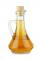Pharmacy

The pharmacy add to 3 liters of 95-percent alcohol 5 liters of 38.04 percent alcohol. How many percent pharmacy got?

Result

p =  59.406 %

Solution:

3*0.95+5*0.3805 = p/100 *(3+5)

8p = 475.25

p = 190132 = 59.40625

Calculated by our simple equation calculator.

Leave us a comment of example and its solution (i.e. if it is still somewhat unclear...):Be the first to comment!To solve this example are needed these knowledge from mathematics:

Looking for a statistical calculator? Our percentage calculator will help you quickly calculate various typical tasks with percentages. Do you have a linear equation or system of equations and looking for its solution? Or do you have quadratic equation? Tip: Our volume units converter will help you with converion of volume units.

Next similar examples:

1. ConcentrationHow 0.5 liters of 8% vinegar diluted to a concentration of 20 hundredths % of vinegar? How many liters of water must be pour?
2. Hydrochloric acidDetermine the concentration of which must have a solution of hydrochloric acid that mixing 10 l of the solution with 8 liters of 26% solution to get the solution with a concentration of 50%?
3. The ballThe ball was discounted by 10 percent and then again by 30 percent. How many percent of the original price is now?Calculate how many percent will increase the length of an HTML document, if any ASCII character unnecessarily encoded as hexadecimal HTML entity composed of six characters (ampersand, grid #, x, two hex digits and the semicolon). Ie. space as: &#x20;
5. PercentCalculate how many % is the number 26.25 less than the number 105.
6. Percentages52 is what percent of 93?
7. PersonsPersons surveyed:100 with result: Volleyball=15% Baseball=9% Sepak Takraw=8% Pingpong=8% Basketball=60% Find the average how many like Basketball and Volleyball. Please show your solution.
8. PercentsHow many percents is 900 greater than the number 750?
9. Jane classWhen asked how many students are in class, Jane said, if we increase the number of students in our class by hundred % and then add half the number of students, we get 100. How many students are in Jane's class?
10. Cinema ticketsCinema sold 180 tickets this Thursday, which is 20%. Monday 14%, Tuesday 6%, Wednesday 9%, Friday 24%, Saturday 12%, and Sunday 15%. How many tickets were sold per week?
11. PupilsThere are 350 girls in the school, and the other 30% of the total number of pupils are boys. How many pupils does the school have?
12. Reducing numberReducing the an unknown number by 28.5% we get number 243.1. Determine unknown number.
13. Perctentages35% of what number is 35?
14. Profit gainIf 5% more is gained by selling an article for Rs. 350 than by selling it for Rs. 340, the cost of the article is:
15. PineHow much of a mixed forest rangers want to cut down, if their head said: "We only cut down pine trees, which in our mixed forest 98%. After cut pine trees constitute 94% of all trees left."
16. Fifth of the numberThe fifth of the number is by 24 less than that number. What is the number?
17. Theorem proveWe want to prove the sentence: If the natural number n is divisible by six, then n is divisible by three. From what assumption we started?